Next: MINIMUM DOMINATING SET Up: Covering and Partitioning Previous: Covering and Partitioning   Index

### MINIMUM VERTEX COVER

• INSTANCE: Graph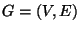.
• SOLUTION: A vertex cover for G, i.e., a subsetsuch that, for each edge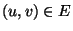, at least one of u and v belongs to V'.
• MEASURE: Cardinality of the vertex cover, i.e.,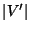.

• Good News: Approximable within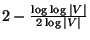and  and.
• Bad News: Not approximable within 1.1666 .
• Comment: The good news hold also for the weighted version [62,232], in which each vertex has a nonnegative weight and the objective is to minimize the total weight of the vertex cover. Admits a PTAS for planar graphs  and for unit disk graphs , even in the weighted case. The case when degrees are bounded byis APX-complete  and , for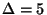not approximable within 1.0029 , and not approximable within 1.1666 for a sufficiently large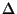. Forit is approximable within 7/6 , and for generalwithin. Approximable within 3/2 for 6-claw-free graphs (where no independent set of size 6 exists in any neighbour set to any vertex) , and within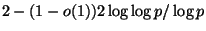for p+1-claw-free graphs . Variation in whichis APX-complete , and is approximable within. Approximable withinif every vertex has degree at leastand . When generalized to k-uniform hypergraphs, the problem is equivalent to MINIMUM SET COVER with fixed number k of occurrences of each elements. Approximable withinand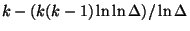, for large values of n and. If the vertex cover is required to induce a connected graph, the problem is approximable within 2 . If the graph is edge-weighted, the solution is a closed walk whose vertices form a vertex cover, and the objective is to minimize the sum of the edges in the cycle, the problem is approximable within 5.5 . The constrained variation in which the input is extended with a positive integer k and a subset S of V, and the problem is to find the vertex cover of size k that contains the largest number of vertices from S, is not approximable withinfor some. The maximization variation in which the input is extended just with a positive integer k, and the problem is to find k vertices that cover as many edges as possible, is 2-approximable  and does not admit a PTAS .
• Garey and Johnson: GT1Next: MINIMUM DOMINATING SET Up: Covering and Partitioning Previous: Covering and Partitioning   Index
Viggo Kann
2000-03-20